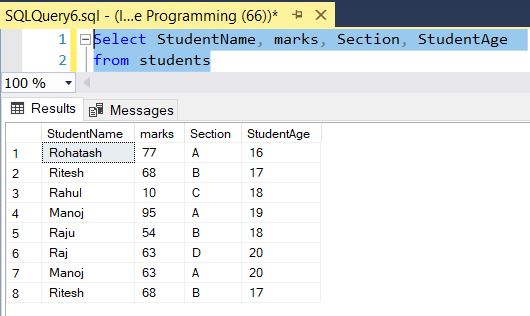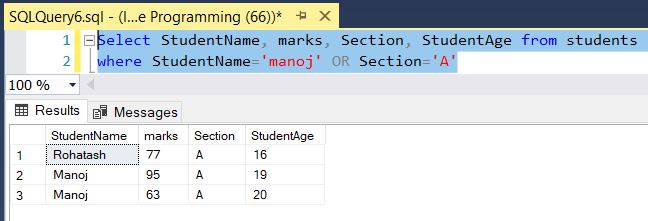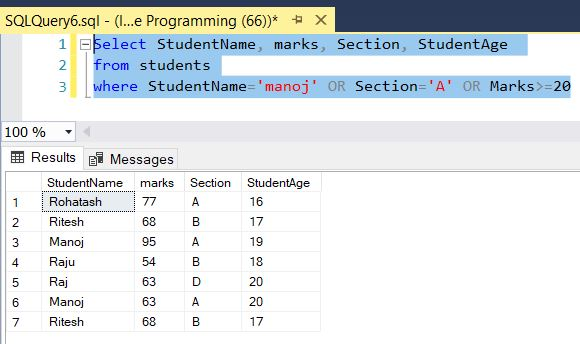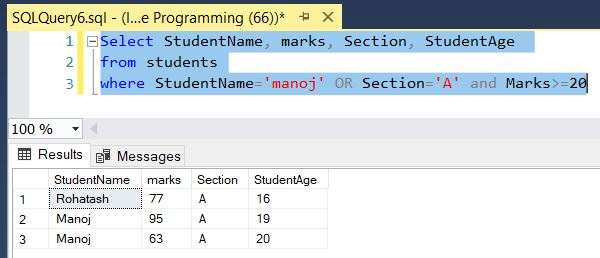﻿ SQL OR Operator - TutorialsTrend
This Angular tutorial helps you get started with Angular quickly and effectively through many practical examples.
`

# SQL-OR Operator

#### SQL Server OR Operator

In many programming languages OR is represented by ||. The OR logical operator is used to filter records based on more than one condition. The OR operator allows you to combine two boolean expressions. It returns TRUE only when one expressions evaluate to TRUE. The AND operator displays a record if any one condition separated by OR are TRUE. The OR operator in SQL is used with the WHERE or HAVING clause.

The following illustrates the syntax of the OR operator:

boolean_expression OR boolean_expression

It is used to check whether the one condition of both side of the operator is true.

The table looks like that:#### OR Operator Example

The SQL command selects StudentName, marks, Section and StudentAge of all students if any one condition matched, where the student name is MANOJ or SECTION as A from the Students table.

``````Select StudentName, marks, Section, StudentAge from students
where StudentName='manoj' OR Section='A'``````

The result is as follows:#### Multiple OR Operators Example

The following statement finds the students that meet all the following conditions:

``````Select StudentName, marks, Section, StudentAge
from students
where StudentName='manoj' OR Section='A' OR Marks>=20``````

Here, The SQL command selects StudentName, marks, Section and StudentAge of all students if any one condition matched, where the student name is MANOJ, SECTION as A or marks greater than equal 20 from the Students table.

The result is as follows:#### OR Operator with Other Logical Operators

In this example, we used both OR and AND operators in the condition. As always, SQL Server evaluated the AND operator first. Therefore, the query retrieved the students whose Section is A and marks is greater than 20 or those whose Studentname is MANOJ.

``````Select StudentName, marks, Section, StudentAge
from students
where StudentName='manoj' OR Section='A' and Marks>=20``````

The result is as follows:To select the student where the student name is MANOJ and section as A and marks is greater than 20 you use parentheses as follows:

``````Select StudentName, marks, Section, StudentAge
from students
where (StudentName='manoj' OR Section='A') and Marks>=20``````

Next